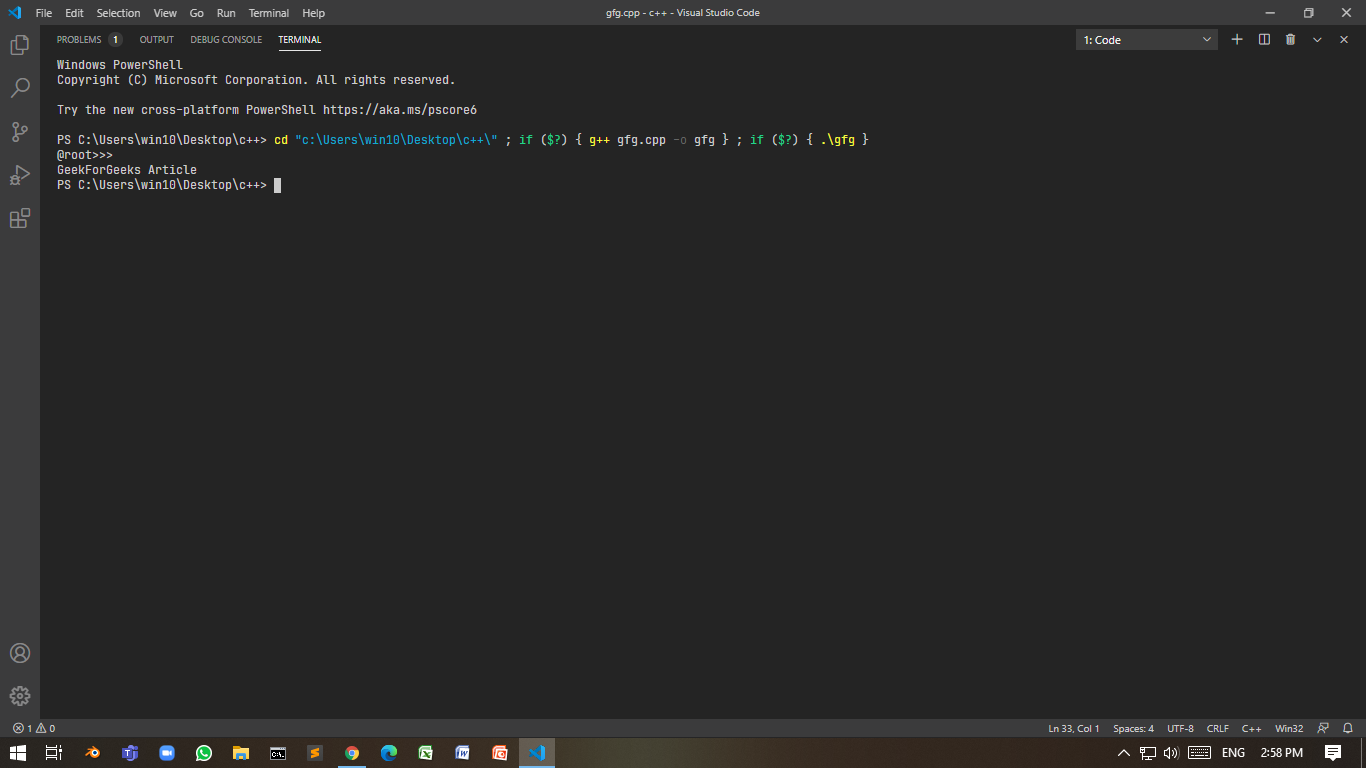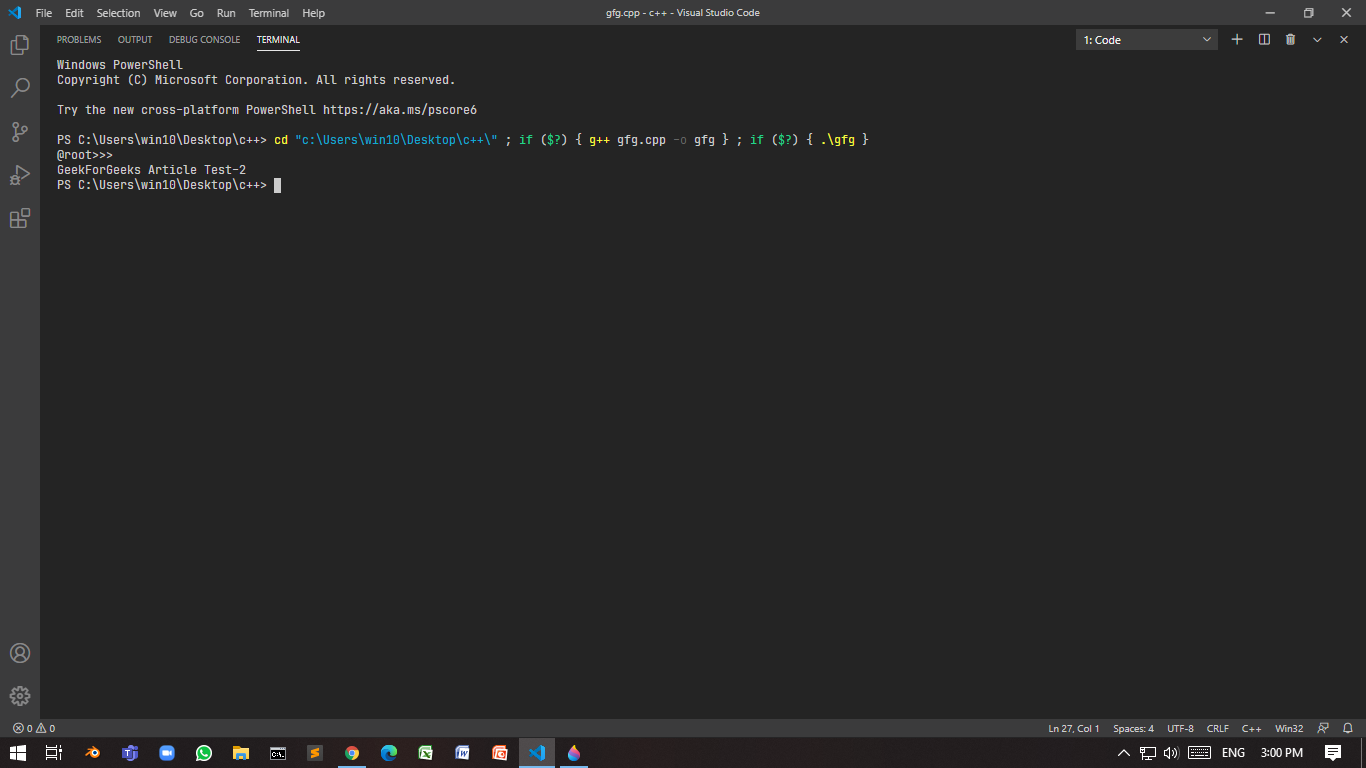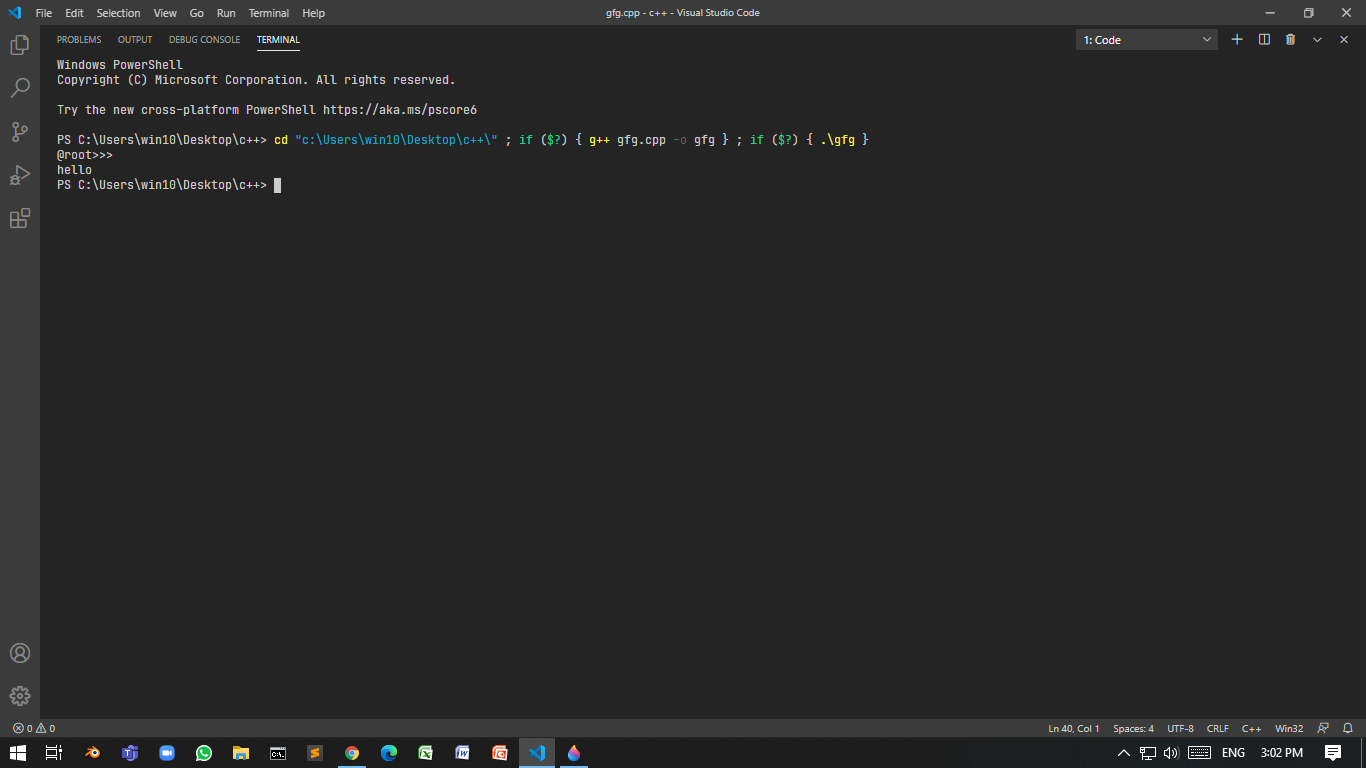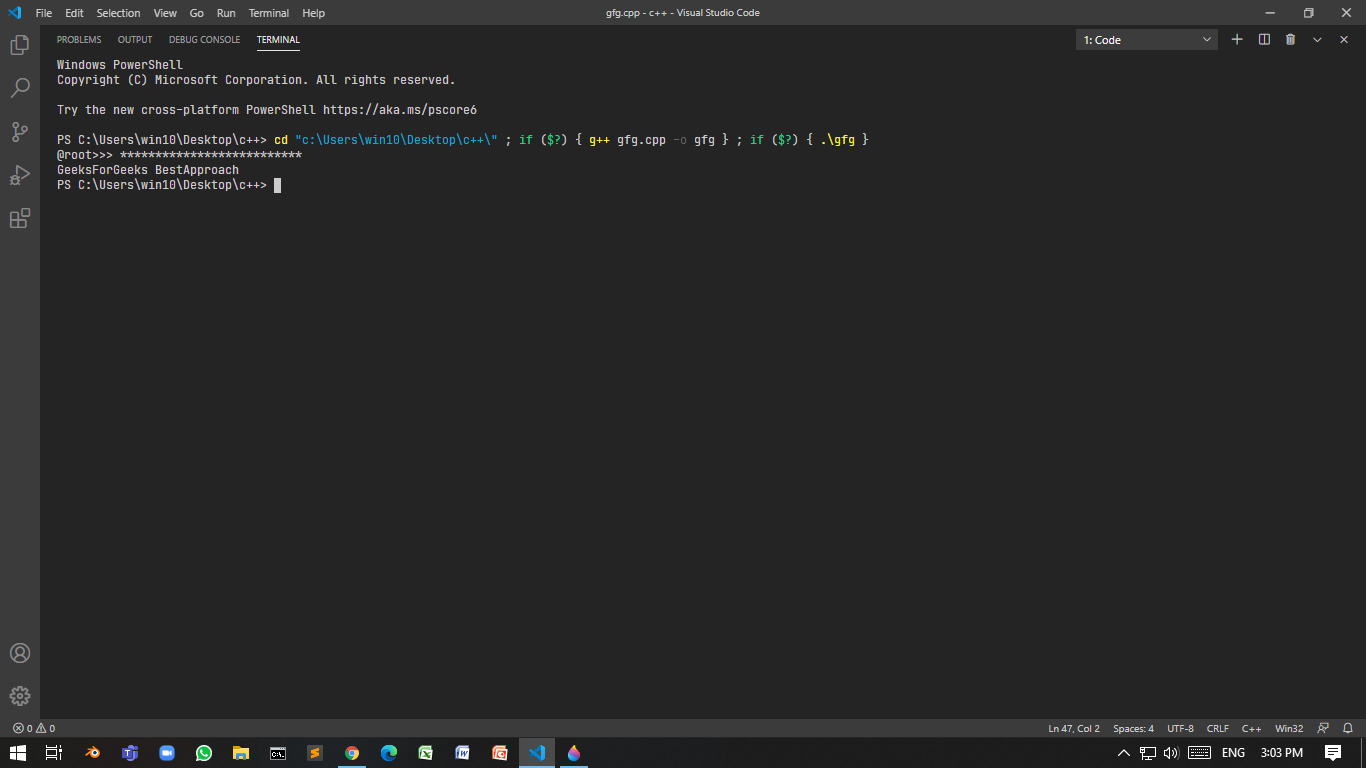Open In App

# Taking password as input in C++

There are two methods in which input can be taken in a more secure way:

• Do not display any content.
• Display a special character such as an asterisk instead of actual content.

In this method, input content will be invisible. This can be implemented in two ways:

### using <windows.h>:

Program 1:

Below is the program where console mode is set to enable, echo input, and reset the console mode:

## C++

 `// C++ program to take the input``// invisibly``#include ``#include ``using` `namespace` `std;`` ` `// Function take password and``// reset to console mode``std::string takePasswdFromUser()``{``    ``HANDLE` `hStdInput``        ``= GetStdHandle(STD_INPUT_HANDLE);``    ``DWORD` `mode = 0;`` ` `    ``// Create a restore point Mode``    ``// is know 503``    ``GetConsoleMode(hStdInput, &mode);`` ` `    ``// Enable echo input``    ``// set to 499``    ``SetConsoleMode(``        ``hStdInput,``        ``mode & (~ENABLE_ECHO_INPUT));`` ` `    ``// Take input``    ``string ipt;``    ``getline(cin, ipt);`` ` `    ``// Otherwise next cout will print``    ``// into the same line``    ``cout << endl;`` ` `    ``// Restore the mode``    ``SetConsoleMode(hStdInput, mode);`` ` `    ``return` `ipt;``}`` ` `// Driver Code``int` `main()``{``    ``string input;``    ``cout << ``"@root>>> "``;`` ` `    ``// Function Call``    ``input = takePasswdFromUser();`` ` `    ``// Print the input``    ``cout << input << endl;``}`

Output:### using <conio.h>:

For this getch() is used. This function takes a character input from user without buffer and doesn’t wait for the user to press “return” key.

Program 2:

Below is the C++ program to demonstrate the use of getch() in conio.h:

## C++

 `// C++ program to demonstrate the``// use of getch()``#include ``#include ``using` `namespace` `std;`` ` `// Function using getch()``std::string takePasswdFromUser()``{``    ``string ipt = ``""``;``    ``char` `ipt_ch;``    ``while` `(``true``) {``        ``ipt_ch = getch();`` ` `        ``// Check whether user enters``        ``// a special non-printable``        ``// character``        ``if` `(ipt_ch < 32) {``            ``cout << endl;``            ``return` `ipt;``        ``}``        ``ipt.push_back(ipt_ch);``    ``}``}`` ` `// Driver Code``int` `main()``{``    ``string input;``    ``cout << ``"@root>>> "``;`` ` `    ``// Function call``    ``input = takePasswdFromUser();``    ``cout << input << endl;``}`

Output:Drawback: The user can’t clear the response made earlier. When backspace is pressed, the input is returned.

Program 3:

Below is the C++ program to demonstrate the solution to the above drawback:

## C++

 `// C++ program to demonstrate the``// solution of above drawback``#include ``#include ``using` `namespace` `std;`` ` `// Enumerator``enum` `TT_Input {`` ` `    ``// ASCII code of backspace is 8``    ``BACKSPACE = 8,``    ``RETURN = 32``};`` ` `// Function accepting password``std::string takePasswdFromUser()``{``    ``string ipt = ``""``;``    ``char` `ipt_ch;``    ``while` `(``true``) {``        ``ipt_ch = getch();`` ` `        ``if` `(ipt_ch < TT_Input::RETURN``            ``&& ipt_ch != TT_Input::BACKSPACE) {``            ``cout << endl;``            ``return` `ipt;``        ``}`` ` `        ``// Check whether the user``        ``// pressed backspace``        ``if` `(ipt_ch == TT_Input::BACKSPACE) {`` ` `            ``// Check if ipt is empty or not``            ``if` `(ipt.length() == 0)``                ``continue``;``            ``else` `{`` ` `                ``// Removes last character``                ``ipt.pop_back();`` ` `                ``continue``;``            ``}``        ``}``        ``ipt.push_back(ipt_ch);``    ``}``}`` ` `// Driver Code``int` `main()``{``    ``string input;``    ``cout << ``"@root>>> "``;`` ` `    ``// Function call``    ``input = takePasswdFromUser();``    ``cout << input << endl;``}`### Hiding the password by a special character(*):

The idea is to use the library <conio.h> here to hide password with asterisk(*). Below is the C++ program using conio.h to hide the password using *:

Program 4:

## C++

 `// C++ program to hide the password``// using *(asterik)``#include ``#include ``using` `namespace` `std;`` ` `// Enumerator``enum` `IN {`` ` `    ``// 13 is ASCII for carriage``    ``// return``    ``IN_BACK = 8,``    ``IN_RET = 13`` ` `};`` ` `// Function that accepts the password``std::string takePasswdFromUser(``    ``char` `sp = ``'*'``)``{``    ``// Stores the password``    ``string passwd = ``""``;``    ``char` `ch_ipt;`` ` `    ``// Until condition is true``    ``while` `(``true``) {`` ` `        ``ch_ipt = getch();`` ` `        ``// if the ch_ipt``        ``if` `(ch_ipt == IN::IN_RET) {``            ``cout << endl;``            ``return` `passwd;``        ``}``        ``else` `if` `(ch_ipt == IN::IN_BACK``                 ``&& passwd.length() != 0) {``            ``passwd.pop_back();`` ` `            ``// Cout statement is very``            ``// important as it will erase``            ``// previously printed character``            ``cout << ``"\b \b"``;`` ` `            ``continue``;``        ``}`` ` `        ``// Without using this, program``        ``// will crash as \b can't be``        ``// print in beginning of line``        ``else` `if` `(ch_ipt == IN::IN_BACK``                 ``&& passwd.length() == 0) {``            ``continue``;``        ``}`` ` `        ``passwd.push_back(ch_ipt);``        ``cout << sp;``    ``}``}`` ` `// Driver Code``int` `main()``{``    ``string input;``    ``cout << ``"@root>>> "``;`` ` `    ``// Function call``    ``input = takePasswdFromUser();``    ``cout << input << endl;``}`